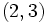# Pronormality is not centralizer-closed

This article gives the statement, and possibly proof, of a subgroup property (i.e., pronormal subgroup) not satisfying a subgroup metaproperty (i.e., centralizer-closed subgroup property).
View all subgroup metaproperty dissatisfactions | View all subgroup metaproperty satisfactions|Get help on looking up metaproperty (dis)satisfactions for subgroup properties
Get more facts about pronormal subgroup|Get more facts about centralizer-closed subgroup property|

## Statement

It is possible to have a group$G$ and a pronormal subgroup$H$ of$G$ such that the centralizer$C_G(H)$ is not pronormal.

## Proof

### Example of the symmetric group

Further information: symmetric group:S4, dihedral group:D8

Suppose$G$ is the symmetric group on the set$\{ 1,2,3,4 \}$ and$H$ is a$2$-Sylow subgroup of$G$, given by:$H = \{ (), (1,2,3,4), (1,3)(2,4), (1,4,3,2), (1,3), (2,4), (1,2)(3,4), (1,4)(2,3) \}$.

Then,$H$ is a pronormal subgroup of$G$, because it is a Sylow subgroup and Sylow implies pronormal. On the other hand, we have:$C_G(H) = \{ (), (1,3)(2,4) \}$.

This is not pronormal, because it is conjugate to the subgroup$\{ (), (1,2)(3,4) \}$ via the permutation$(2,3)$, but these are not conjugate in the subgroup they generate.

Let$G$ be the symmetric group on the set$\{ 1,2,3,4 \}$.